# To analyse whether the given statement: tert-Butylamine is 3 0 amine, is true or not. Concept Introduction: Amines are the derivatives of ammonia, wherein one or more than one hydrogen atoms are substituted by an alkyl or aryl group. The amines are categorized as primary, secondary or tertiary based on the number of carbon atoms that are bonded directly to the nitrogen atom. Primary amine has only one carbon atom bonded to the nitrogen atom. Similarly, secondary amines have two carbon groups bonded to the nitrogen, and tertiary amines nave three carbon groups bonded to the nitrogen.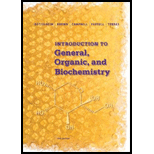### Introduction to General, Organic a...

11th Edition
Frederick A. Bettelheim + 4 others
Publisher: Cengage Learning
ISBN: 9781285869759### Introduction to General, Organic a...

11th Edition
Frederick A. Bettelheim + 4 others
Publisher: Cengage Learning
ISBN: 9781285869759

#### Solutions

Chapter 16, Problem 16.6P

(a)

Interpretation Introduction

## Interpretation: To analyse whether the given statement: tert-Butylamine is 30 amine, is true or not.Concept Introduction:Amines are the derivatives of ammonia, wherein one or more than one hydrogen atoms are substituted by an alkyl or aryl group.The amines are categorized as primary, secondary or tertiary based on the number of carbon atoms that are bonded directly to the nitrogen atom. Primary amine has only one carbon atom bonded to the nitrogen atom. Similarly, secondary amines have two carbon groups bonded to the nitrogen, and tertiary amines nave three carbon groups bonded to the nitrogen.

Expert Solution

The statement is false.

### Explanation of Solution

The structure of a tert-butylamine is given below: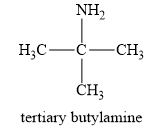In a tert-butylamine, the carbon atom is bonded to three methyl groups, while nitrogen is attached to only one carbon group. Therefore, the tert-butyl amine is a primary amine. Therefore, this statement is false.

(b)

Interpretation Introduction

### Interpretation: To analyse whether the given statement about the aromatic amine is true or not.Concept Introduction:Amines are the derivatives of ammonia, wherein one or more than one hydrogen atoms are substituted by an alkyl or aryl group.The amines are categorized as primary, secondary or tertiary based on the number of carbon atoms that are bonded directly to the nitrogen atom. Primary amine has only one carbon atom bonded to the nitrogen atom. Similarly, secondary amines have two carbon groups bonded to the nitrogen, and tertiary amines nave three carbon groups bonded to the nitrogen.

Expert Solution

The statement is true.

### Explanation of Solution

If in an amine, the nitrogen is directly bonded to one or more aryl groups or aromatic rings, and then the amine is known as an aromatic amine.

For example, aniline is an aromatic amine, as it is attached to one aromatic ring benzene. The structure of aniline is given below: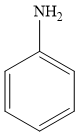Therefore, the given statement is true.

(c)

Interpretation Introduction

### Interpretation: To analyse whether the given statement: In a heterocyclic amine, the main nitrogen is the part of the ringis true or not.Concept Introduction:Amines are the derivatives of ammonia, wherein one or more than one hydrogen atoms are substituted by an alkyl or aryl group.The amines are categorized as primary, secondary or tertiary based on the number of carbon atoms that are bonded directly to the nitrogen atom. Primary amine has only one carbon atom bonded to the nitrogen atom. Similarly, secondary amines have two carbon groups bonded to the nitrogen, and tertiary amines nave three carbon groups bonded to the nitrogen.

Expert Solution

The statement is true.

### Explanation of Solution

In a heterocyclic amine, the carbon group of the ring structure is replaced by the nitrogen atom. For example, in pyridine, the nitrogen atom replaces one —CH group of the benzene ring.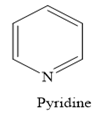Thus, the heterocyclic amine is an amine in which nitrogen is one of the atoms of a ring. Therefore, this statement is true.

(d)

Interpretation Introduction

### Interpretation: To analyze whether the given statement: The NH4+ and CH4have the same number of valence electrons and both have tetrahedral geometry as per the VSEPR model is true or not.Concept Introduction:The VSEPR (Valence-shell electron pair repulsion) model predicts the shape of the molecules or ions by identifying the position of atoms connected to the central atom.

Expert Solution

The statement is true.

### Explanation of Solution

The valence shell electron pair repulsion (VSEPR) theory determines the shapes and the geometry of a molecule. In a CH4 molecule, one carbon atom is bonded to four hydrogen atoms with a single bond. In NH4+, one nitrogen atom is singly bonded to four hydrogen atoms.

According to the VSEPR model, each bond represents a pair of electrons. Since both compounds have four bonds around the central atom, they contain eight valence electrons. Also, according to the VSEPR model, these four bonding regions are arranged in a tetrahedral manner so that they are as far away from one another as possible, giving the molecule a tetrahedral geometry. Therefore, this statement is true.

(e)

Interpretation Introduction

### Interpretation: To analyze whether the given statement: There are four constitutional isomers with the molecular formulaC3H9N, is true or not.Concept Introduction:Constitutional isomers are those compounds which have same molecular formula, but they differ in the arrangement of atoms in the molecules.

Expert Solution

The statement is true.

### Explanation of Solution

The molecular formula is given as C3H9N. Therefore, the possible constitutional isomers for this molecular formula are given below: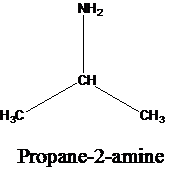Thus, there are four constitutional isomers possible with the molecular formula C3H9N. Therefore, this statement is true.

### Want to see more full solutions like this?

Subscribe now to access step-by-step solutions to millions of textbook problems written by subject matter experts!

### Want to see more full solutions like this?

Subscribe now to access step-by-step solutions to millions of textbook problems written by subject matter experts!